### Transformers Everywhere - Patch Encoding Technique for Vision Transformers(ViT) Explained

Posted December 24, 2021 by Gowri Shankar  ‐  8 min read

Today, we are witnessing transformer architecture making the breakthrough in almost all the AI challenges because of its domain agnostic nature and simplicity. For language models, transformers are the single stop default solution for convergence, then transformers are applied for time series forecasting(Temporal Fusion Transformers). The key concepts of self-attention and positional encoding make the fundamental building blocks of transformer architecture, that can be extended to images as well. Dosovitsky, Kolesnivkov et al of Google has demonstrated that an image can be represented completely with [16 x 16] words in their 2021 paper titled _An image is worth 16 x 16 Words: Transformers for Image Recognition at Scale_. That means the most reliable CNN architecture has a partner now to take computer vision solutions to their new heights.

This is the $5^{th}$ post in transformer series where we are focusing on the how to process and feed the input data into a transformer. The previous posts in transformer series can be referred in the following links,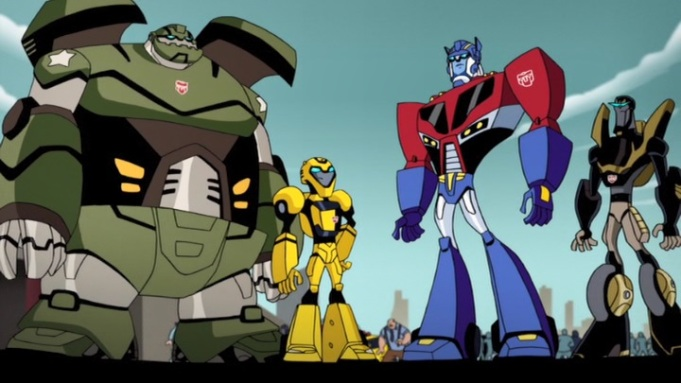This post is a walk-through of the paper titled An image is worth 16 x 16 Words: Transformers for Image Recognition at Scale from Google Research and Brain. I thank Yannic Kilcher, Prajit Ramachandran, Sayak Paul, Khalid Salama, and Aravind Srinivas for their detailed explanation of ViT in Keras portal, ICML2021, and various other sources. Without their quality work, this post wouldn’t have been possible.

### Objective

Objective of this post is to learn input representation scheme of Vision Transformers.

## Introduction

Subsequently, positional encoding is incorporated with an equal number of vectors($d$) to predict the position of a word in the sequence. This luxury is primarily impossible for images because of a simple reason - the unit representation of an image is pixels and there are too many pixels in an image when we compared to the number of words in a sentence. The core of the attention model is the classic matrix multiplication of Query(Q) and the Key(K) $(QK^T)$ is a quadratic operation. An image having $224 \times 224 \times 3 \Rightarrow heights \times width \times channels$ dimension will result in occupying trillions of bytes in the memory for a small dataset - which is primarily not possible even for modern-day hardware.

### Compute Complexity

The $Q\odot K^T$ operation looks computationally quite daunting because we are multiplying every element of the matrix that lead to $O(n^2.d)$ complexity. Where $n$ is the length of the sequence and $d$ is the representation dimension. Meanwhile, an attention model does not have any recurrent or convolution layers, making it a mere positional connection with a constant number of sequentially executed operations.

In NLP, It is a rare event where the length of the sequence$(n)$ exceeds the representation dimension$(d)$, ensuring the total computational complexity of the attention mechanism is far lesser than a recurrent model with a per layer complexity leads to $O(n.d^2)$. Complexity further reduces when the self-attention mechanism restricts the size of the query with a focused set of input tokens.

On contrary, in vision models $n$ is far greater($224 \times 224 \times 3 = 150,528$) than the representation dimension $(d)$. $$i.e$$ $$x \in \mathbb{R}^n \ vs \ x \in \mathbb{R}^{H \times W \times C}$$ $$where, \ n « H \times W \times C$$

### Math Behind Image Patches

The images split into multiple patches of the same height and width $P$. i.e. $$x \in \mathbb{R}^{H \times W \times C} \Rightarrow x \in \mathbb{R}^{N \times P^2 C} \tag{2. Image to Patch Transformation}$$

Where,

• $N = \frac{HW}{P^2}$ is the number of patches
• $P$ is the height and width of the patch with a patch dimension $(P, P, C)$
• $C$ is the number of channels

The image patches section demonstrates the patch creation scheme in detail.

### Image to Flattened Image Patches

Following code makes image patches from an input image.

• Reads the image from the disk
• Converts the image object to TensorFlow array
• Crops the input image to multiple of 16 pixels, where patch size $P=16$
• Extracts the patches using TensorFlow API
• By keeping the strides equal to patch size $P$, overlapping is avoided
import tensorflow as tf

# Image preprocessing

def read_image(image_file="transformers.jpeg", scale=False, image_dim=336):

image_file, grayscale=False, color_mode='rgb', target_size=None,
interpolation='nearest'
)
image_arr_orig = tf.keras.preprocessing.image.img_to_array(image)
if(scale):
image_arr_orig = tf.image.resize(
image_arr_orig, [image_dim, image_dim],
method=tf.image.ResizeMethod.BILINEAR, preserve_aspect_ratio=False
)
image_arr = tf.image.crop_to_bounding_box(
image_arr_orig, 0, 0, image_dim, image_dim
)

return image_arr

# Patching
def create_patches(image):
im = tf.expand_dims(image, axis=0)
patches = tf.image.extract_patches(
images=im,
sizes=[1, 16, 16, 1],
strides=[1, 16, 16, 1],
rates=[1, 1, 1, 1],
)
patch_dims = patches.shape[-1]
patches = tf.reshape(patches, [1, -1, patch_dims])

return patches

patches = create_patches(image_arr)

2021-12-24 12:41:26.513461: I tensorflow/core/platform/cpu_feature_guard.cc:151] This TensorFlow binary is optimized with oneAPI Deep Neural Network Library (oneDNN) to use the following CPU instructions in performance-critical operations:  AVX2 FMA
To enable them in other operations, rebuild TensorFlow with the appropriate compiler flags.

# Drawing
import numpy as np
import matplotlib.pyplot as plt

def render_image_and_patches(image, patches):
plt.figure(figsize=(8, 8))
plt.suptitle(f"Cropped Image", size=24)
plt.imshow(tf.cast(image, tf.uint8))
plt.axis("off")
n = int(np.sqrt(patches.shape))
plt.figure(figsize=(8, 8))
plt.suptitle(f"Image Patches", size=24)
for i, patch in enumerate(patches):
ax = plt.subplot(n, n, i+1)
patch_img = tf.reshape(patch, (16, 16, 3))
ax.imshow(patch_img.numpy().astype("uint8"))
ax.axis("off")

def render_flat(patches):
plt.figure(figsize=(32, 2))
plt.suptitle(f"Flattened Image Patches", size=24)
n = int(np.sqrt(patches.shape))
for i, patch in enumerate(patches):
ax = plt.subplot(1, 24, i+1)
patch_img = tf.reshape(patch, (16, 16, 3))
ax.imshow(patch_img.numpy().astype("uint8"))
ax.axis("off")
if(i == 23):
break

render_image_and_patches(image_arr, patches)
render_flat(patches)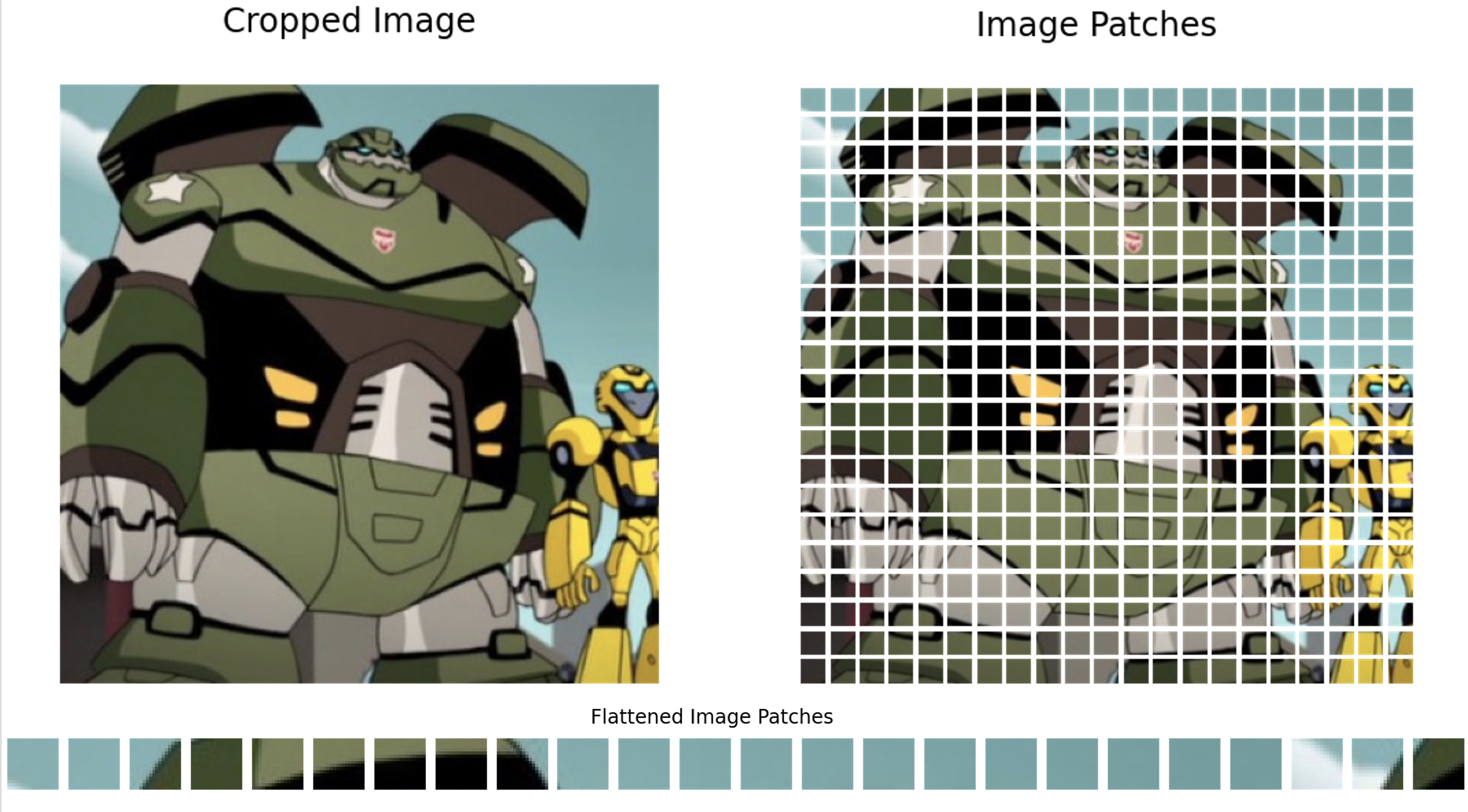These fixed size patches are flattened and projected linearly to embed each of them with positional encoding. The interesting part of vision transformer is, once the positional encoding is done - the embedded sequence is fed into the standard transformer. We shall see this in action in the next section.

## ViT Architecture

The only difference between the transformers of NLP and ViT is the way we treat the input data. i.e We have embeddings of tokenized words for language processing and linearly projected images patches for vision transformers. More on self attention and positional encoding can be found here,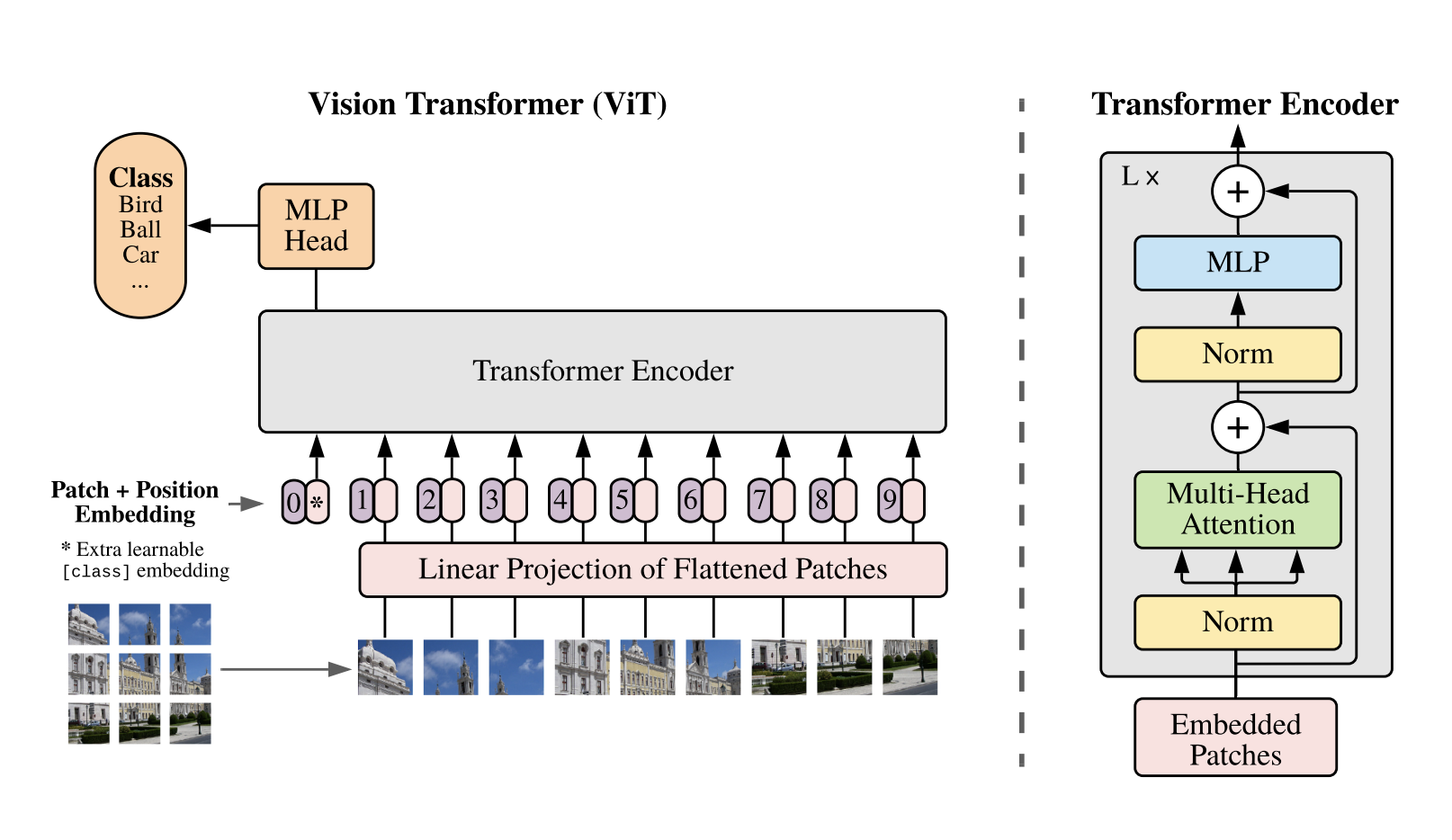The above diagram renders 3 important aspects of the visual transformers,

• The way image patches are sequenced and fed into the system
• The linear projection of flattened patches
• Patch + Position Embedding(similar to transformer encoder of Vaswani et al) with an extra learnable embedding entity that determines the class of the image

In the subsequent sections, let us dissect the internals of the linear projection and patch encoding in an intuitive way.

### Patch Embedding

Patch embedding takes inspiration from the BERT architecture on tokenizing the input sequences. i.e. Prepending the class information to the sequence of embedded patches, $$z_0^0 = x_{class} \Rightarrow z_L^0 = y \tag{3. Image Classes}$$



The Transformer uses constant latent vector size D
through all of its layers, so we flatten the patches
and map to D dimensions with a trainable linear
projection.

To perform classification, we use the standard approach of adding an extra learnable “classification token”
to the sequence.

- Dosovitskiy et al



During pre-training and fine-tuning, a classification head is attached to $z_L^0$ and the classification is by a multi-layer perceptron(with $GELU$) with one hidden layer at pre-training time.

$$z_0 =[x_{class}; x_p^1E; x_p^2E; \cdots, x_p^NE] + E_{pos}, E \in \mathbb{R}^{(P^2.C) \times D}, E_{pos} \in \mathbb{R}^{(N+1) \times D} \tag{4. Patch Encoding}$$

### Linear Projection and Position Encoding

We took a significantly large image to understand the patch creation and flattening process. In this section, let us scale the image to the size $(64 \times 64)$ and create embeddings. Three steps,

1. Linear projection - using a Dense layer
2. Represent it using a lower-dimensional vector - using an Embedding layer and
3. Finally, sum them both.

This section does not incorporate the extra learnable parameter of $z_0$ for simplicity

NUM_PATCHES = 16
PROJECTION_DIM = 4

image_arr = read_image(image_file="t2.png", scale=True, image_dim=64)
patches = create_patches(image_arr)

positions = tf.range(start=0, limit=NUM_PATCHES, delta=1)
projection = tf.keras.layers.Dense(units=PROJECTION_DIM)(patches)
position_embedding = tf.keras.layers.Embedding(input_dim=NUM_PATCHES, output_dim=PROJECTION_DIM)(positions)

final_embedding = projection + position_embedding


Let us examine the shape of the embedding and the patches.

orig_size = np.prod(patches.shape)
size_representation = np.prod(final_embedding.shape)
print(f"Shape of patches: {patches.shape} ⇒ {orig_size}, Shape of the final embedding: {final_embedding.shape} ⇒ {size_representation}")
print(f"1:{abs(orig_size / size_representation)} time reduced")

Shape of patches: (1, 16, 768) ⇒ 12288, Shape of the final embedding: (1, 16, 4) ⇒ 64
1:192.0 time reduced

render_image_and_patches(image_arr, patches)
render_flat(patches)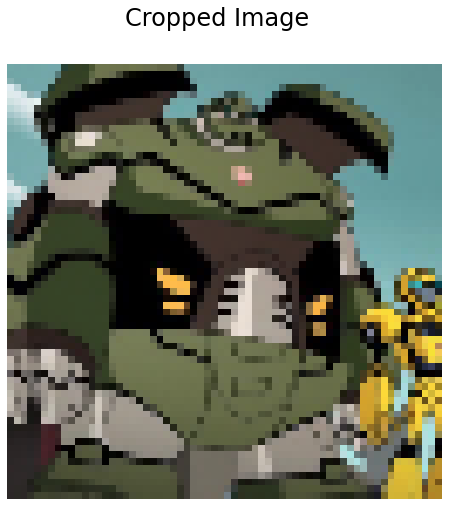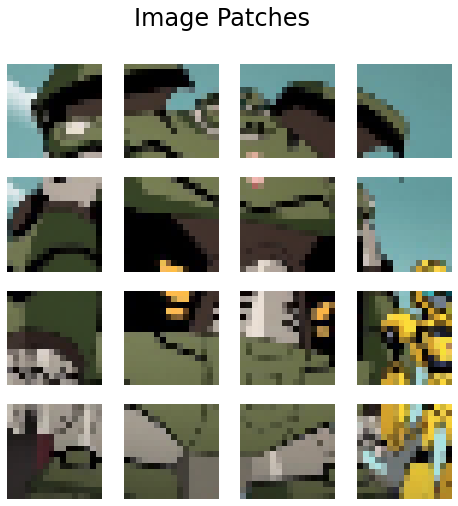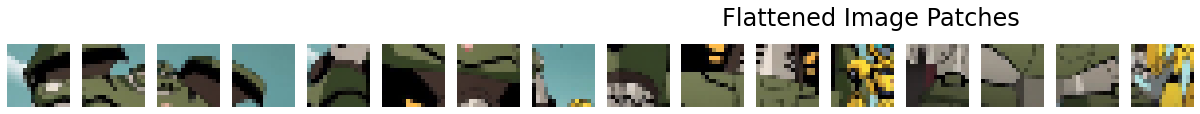## Epilogue

In this post, we studied how Vision Transformers work by focusing on the Patch Encoding scheme of input representation. We have consummated a significant amount of study materials dealing with the fundamentals of transformer architecture, Now we have the relevant tools and intuition to expand our horizons on going much deeper into transformers and attention. Following are a few of the important breakthroughs in transformers that one might have to consider exploring,

Hope you enjoyed reading this post. Wishing Merry Christman and Happy New Year to all.

#transformers-everywhere-patch-encoding-technique-for-vision-transformersvit-explained